6 Jul 2013

The Wiener filter is well known as the optimal solution to the problem of estimating a random process when it is corrupted by another additive process, using only a linear combination of values of the measured process. Mathematically, this means that the Wiener filter constructs an estimator of some original signal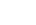given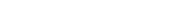with the property that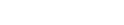is minimized among all such linear estimators, assuming only that bothand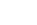are stationary and have known statistics (mean, variance, power spectral density, etc.). When more information about the structure ofis known, different estimators may be easier to implement (such as a Kalman filter for signals with a recursive structure).

Such a filter is very powerful—it is optimal, after all—when the necessary statistics are available and the input signals meet the requirements, but in practice, signals of interest are never stationary (rarely even wide sense stationary, although it is a useful approximation), and their statistics change frequently. Rather than going through the derivation of the filter, which is relatively straightforward and available on Wikipedia (linked above), I'd like to talk about how to adapt it to situations that do not meet the filter’s criteria and still obtain high quality results, and then provide a demonstration on one such signal.

The first problem to deal with is the assumption that a signal is stationary. True to form for engineering, the solution is to look at only a brief portion of the signal and approximate it as stationary. This has the unfortunate consequence of preventing us from defining the filter once and reusing it; instead, as the measured signal is sliced into approximately stationary segments, we must estimate the relevant statistics and construct an appropriate filter for each segment. If we do the filtering in the frequency domain, then for segments of length N we are able to do the entire operation with two length N FFTs (one forward and one reverse) and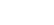arithmetic operations (mostly multiplication and division). This is comparable to other frequency domain filters and much faster than thenumber of operations required for a time domain filter.

This approach creates a tradeoff. Because the signal is not stationary, we want to use short time slices to minimize changes. However, the filter operates by adjusting the amplitude of each bin after a transformation to the frequency domain. Therefore, we want as many bins as possible to afford the filter high resolution. Adjusting the sampling rate does not change the frequency resolution for a given amount of time, because the total time duration of any given buffer is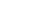. So, for fixed time duration, the length of the buffer will scale inversely with the sampling rate, and the bin spacing in an FFT will remain constant. The tradeoff, then, exists between how long each time slice will be and how much change in signal parameters we wish to tolerate. A longer time slice weakens the stationary approximation, but it also produces better frequency resolution. Both of these affect the quality of the resulting filtered signal.

The second problem is the assumption that the statistics are known beforehand. If we're trying to do general signal identification, or simply "de-noising" of arbitrary incoming data (say, for sample, cleaning up voice recorded from a cell phone's microphone in windy area, or reducing the effects of thermal noise in a data acquisition circuit), then we don't know what the signal will look like beforehand. The solution here is a little bit more subtle. The normal formulation of the Wiener filter, in the Laplace domain, is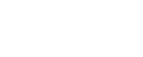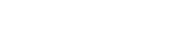In this case we assume that the cross-power spectral density,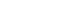, between the measured process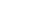and the true processis known, and we assume that the power spectral density,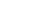, of the measured processis known. In practice, we will estimatefrom measured data, but as the statistics ofare unknown, we don't know whatis (and can't measure it directly). But, we do know the statistics of the noise. And, by (reasonable) assumption, the noise and the signal of interest are independent. Therefore, we can calculate several related spectra and make some substitutions into the definition of the original filter.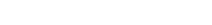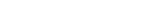If we substitute these into the filter definition to eliminate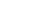, then we are able to construct and approximation of the filter based on the (known) noise PSD and an estimate of the signal PSD (if the signal PSD were known, it'd be exact, but as our PSD estimate contains errors, the filter definition will also contain errors).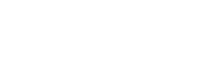You may ask: if we don't know the signal PSD, how can we know the noise PSD? Realistically, we can't. But, because the noise is stationary, we can construct an experiment to measure it once and then use it later. Simply identify a time when it is known that there is no signal present (i.e. ask the user to be silent for a few seconds), measure the noise, and store it as the noise PSD for future use. Adaptive methods can be used to further refine this approach (but are a topic for another day). It is also worth noting that the noise does not need to be Gaussian, nor does it have any other restrictions on its PSD. It only needs to be stationary, additive, and independent of the signal being estimated. You can exploit this to remove other types of interference as well.

One last thing before the demo. Using the PSD to construct the filter like this is subject to a number of caveats. The first is that the variance of each bin in a single PSD estimate is not zero. This is an important result whose consequences merit more detailed study, but the short of it is that the variance of each bin is essentially the same as the variance of each sample from which the PSD was constructed. A remedy for this is to use a more sophisticated method for estimating the PSD by combining multiple more-or-less independent estimates, generally using a windowing function. This reduces the variance and therefore improves the quality of the resulting filter. This, however, has consequences related to the trade-off between time slice length and stationary approximation. Because you must average PSDs computed from (some) different samples in order to reduce the variance, you are effectively using a longer time slice.

Based on the assigned final project in ECE 4110 at Cornell University, which was to use a Wiener filter to de-noise a recording of Einstein explaining the mass-energy equivalence with added Gaussian white noise of unknown power, I've put together a short clip comparing the measured (corrupted) signal, the result after filtering with a single un-windowed PSD estimate to construct the filter, and the result after filtering using two PSD estimates with 50% overlap (and thus an effective length of 1.5x the no-overlap condition) combined with a Hann window to construct the filter. There is a clear improvement in noise rejection using the overlapping PSD estimates, but some of the short vocal transitions are also much more subdued, illustrating the tradeoff very well.

Be warned, the first segment (unfiltered) is quite loud as the noise adds a lot of output power.

Here is the complete MATLAB code used to implement the non-overlapping filter

% Assumes einsteindistort.wav has been loaded with

% Anything that can divide the total number of samples evenly
sampleSize = 512;

% Delete old variables
% clf;
clear input;
clear inputSpectrum;
clear inputPSD;
clear noisePSD;
clear sampleNoise;
clear output;
clear outputSpectrum;
clear weinerCoefficients;

% These regions indicate where I have decided there is a large amount of
% silence, so we can extract the noise parameters here.
noiseRegions = [1 10000;
81000 94000;
149000 160000;
240000 257500;
347500 360000;
485000 499000;
632000 645000;
835000 855000;
917500 937500;
1010000 1025000;
1150000 116500];

% Now iterate over the noise regions and create noise start offsets for
% each one to extract all the possible noise PSDs
noiseStarts = zeros(length(noiseRegions(1,:)), 1);
z = 1;
for k = 1:length(noiseRegions(:,1))
for t = noiseRegions(k,1):sampleSize:noiseRegions(k,2)-sampleSize
noiseStarts(z) = t;
z = z + 1;
end
end

% In an effort to improve the PSD estimate of the noise, average the FFT of
% silent noisy sections in multiple parts of the recording.
noisePSD = zeros(sampleSize, 1);
for n = 1:length(noiseStarts)
sampleNoise = d(noiseStarts(n):noiseStarts(n)+sampleSize-1);
noisePSD = noisePSD + (2/length(noiseStarts)) * abs(fft(sampleNoise)).^2 / sampleSize;
end

% Force the PSD to be flat like white noise, for comparison
% noisePSD = ones(size(noisePSD))*mean(noisePSD);

% Now, break the signal into segments and try to denoise it with a
% noncausal weiner filter.
output = zeros(1, length(d));
for k = 1:length(d)/sampleSize
input = d(1+sampleSize*(k-1):sampleSize*k);
inputSpectrum = fft(input);
inputPSD = abs(inputSpectrum).^2/length(input);
weinerCoefficients = (inputPSD - noisePSD) ./ inputPSD;
weinerCoefficients(weinerCoefficients < 0) = 0;
outputSpectrum = inputSpectrum .* weinerCoefficients;

% Sometimes for small outputs ifft includes an imaginary value
output(1+sampleSize*(k-1):sampleSize*k) = real(ifft(outputSpectrum, 'symmetric'));
end

% Renormalize and write to a file
output = output/max(abs(output));
wavwrite(output, r, 'clean.wav');
</pre>
<p>To convert this implementation to use 50% overlapping filters, replace the filtering loop (below "Now, break the signal into segments…") with this snippet:</p>
<pre class="brush:plain">
output = zeros(1, length(d));
windowFunc = hann(sampleSize);
k=1;
while sampleSize*(k-1)/2 + sampleSize < length(d)
input = d(1+sampleSize*(k-1)/2:sampleSize*(k-1)/2 + sampleSize);
inputSpectrum = fft(input .* windowFunc);
inputPSD = abs(inputSpectrum).^2/length(input);
weinerCoefficients = (inputPSD - noisePSD) ./ inputPSD;
weinerCoefficients(weinerCoefficients < 0) = 0;
outputSpectrum = inputSpectrum .* weinerCoefficients;

% Sometimes for small outputs ifft includes an imaginary value
output(1+sampleSize*(k-1)/2:sampleSize*(k-1)/2 + sampleSize) = output(1+sampleSize*(k-1)/2:sampleSize*(k-1)/2 + sampleSize) + ifft(outputSpectrum, 'symmetric')';
k = k +1;
end


The corrupted source file used for the project can be downloaded here for educational use.

This can be adapted to work with pretty much any signal simply by modifying the noiseRegions matrix, which is used to denote the limits of "no signal" areas to use for constructing a noise estimate.